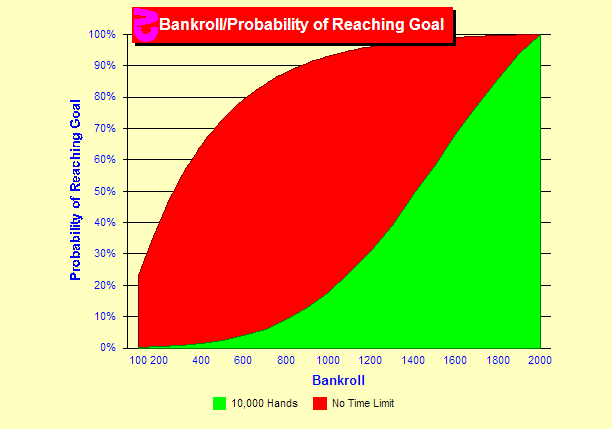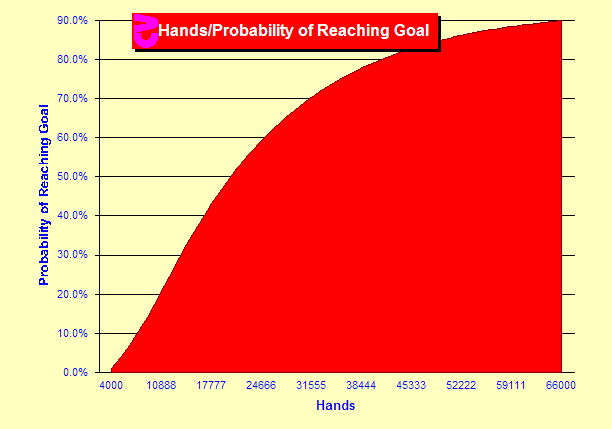# Card Counting Goals

###What is the probability that I will achieve goals?

Let's turn this around and look at the probabilities of success rather than failure. First we will set a goal. In this chart the goal is 2,000 units. The rules, strategy and amounts bet are fixed in all sims on this page and are stated in the Sim Details at the bottom of the page. We now will look at bankrolls from 100 to 2,000 units and plot the probability that we will make this goal. Two curves are provided. The red curve displays the probabilities with no limit on the number of hands. That is we will play until we make our goal or lose our entire bankroll. Obviously if we start at 2,000 units, we have a 100% chance of success since our goal is also 2,000 units. The probability of success rises quickly with larger bankrolls reaching a 50% chance of reaching 2,000 units with a 300 unit bankroll.

The green line is plotted with a time limit in mind. Here we play until we hit our goal, lose our bankroll or play 10,000 hands. The curve is very different indicating that we run out of time often with smaller bankrolls. Even with a bankroll of 1,900 units, we will not hit 2,000 in 10,000 hands about 5% of the time.

###How many hands will it take?

So how many hands must we play? In this chart we still have a goal of 2,000 units, but we fix the bankroll at 1,000 units and look at the probability of reaching the goal at various numbers of hands. At 4,000 hands we have a tiny chance. At 10,000 hands we have a 20% chance of doubling our bankroll, which matches the above chart. The probabilities grow quickly as the time increases and starts leveling off above 30,000 hands. We reach a 90% chance at 66,000 hands.

###What happens when I include a goal and hands?

Let's try varying both the bankroll and the goal. The time limit is fixed at 10,000 hands in this chart. First we will look at a bankroll of 2,000 units. That is the top of the deep blue area in the upper part of this chart. The x-axis is the goal and it is varied from 2,000 to 3,700 units. At the upper left, we see the point for the goal and bankroll both at 2,000 units. Clearly this is a 100% probability. The deep blue line swoops downward in probability as we increase the goal. At the bottom right we see a near zero probability of reaching a goal of 3,700 units in 10,000 hands with the betting levels in these sims.

The different colors display the probabilities given different bankrolls displayed at the bottom of the chart.

Keep in mind that these are just sample charts. Obviously the charts will change dramatically with different games, strategies, bet spreads, time limits, bankrolls and goals. The calculators at Card-Counting.com can be used to calculate new numbers.

### Sim details

• Six decks, S17, DAS, LS, 4.8/6 penetration, 4 players, Hi-Lo, truncate, full indexes, half-deck resolution
• Five billion rounds each, all sims use a fixed betting schedule of 1 to 15 units.